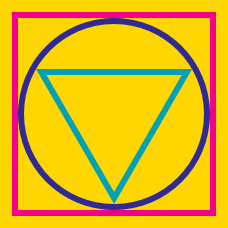Geometry

# Incircle of Squares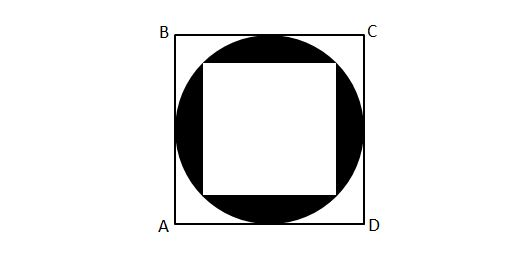In the figure above, the black circle is inscribed in square $\square ABCD,$ and the white square is inscribed in the circle. If the length of $\overline{AB}$ is $\lvert\overline{AB}\rvert=8,$ what is the area of the shaded region?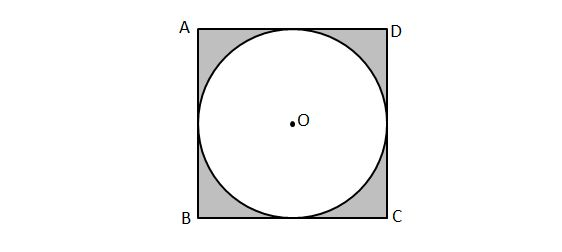In the figure above, circle $O$ of radius $8$ is inscribed in square $ABCD.$ What is the area of the shaded region?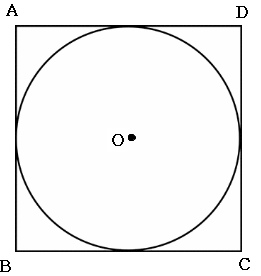Above illustration is a square circumscribed about a circle. If $\overline{CD} = 12,$ What is the area of the circle?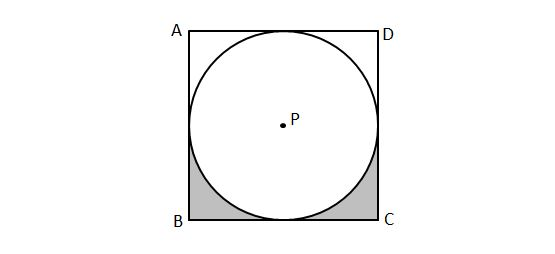In the above diagram, circle $P$ with radius $8$ is inscribed in square $ABCD.$ If a point is picked at random from the interior of the square, what is the probability that the point will lie in the shaded regions?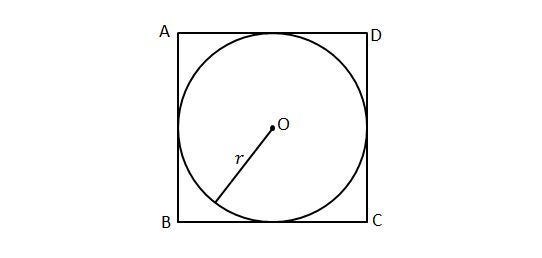In the figure above, if circle $O$ with radius of $5$ is inscribed in square $ABCD$ in such a way that each side of the square is tangent to the circle, which of the following statements must be true?

I. $\lvert{\overline{AB}}\rvert \times \lvert{\overline{CD}}\rvert < 25\pi$
II. $\text{Area of } \square ABCD = 100$
III. $\displaystyle 5 < \frac{2 \times \lvert{\overline{CD}}\rvert}{\pi}$

×